# High School Physics : Understanding Force

## Example Questions

### Example Question #1 : Forces

Two dogs, one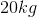and one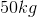, pull on a bone. They each pull with the same force, but in opposite directions. What is the acceleration of the bone?

Possible Answers:

The bone will accelerate towards the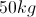dog

The bone will fly into the air

The bone will have positive, then negative acceleration

The bone will have zero acceleration

The bone will accelerate towards the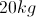dog

Correct answer:

The bone will have zero acceleration

Explanation:

For this problem, we are looking at the net force on the bone. Since both dogs are pulling with the same force, we can say the magnitude of the force of dog 1 equals the magnitude of the force of dog 2, but in opposite directions. Mathematically,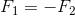.

To find the net force on the bone, we add the individual forces together.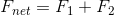We can substitute our forces into the equation for net force.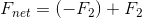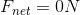The net force is zero. Each dog pulls with equal force in opposite directions, allowing the total force to cancel out.

According to Newton's second law,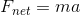. If the net force is zero, then the acceleration of the bone must also be zero.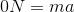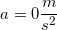### Example Question #2 : Introduction To Forces

A man is painting a house. He notices that there is a small drop of paint that is remaining perfectly still on the vertical wall. What conclusion can he draw about the paint?

Possible Answers:

The paint is water based

We need to know the density of the drop to draw any conclusions

We need to know the mass of the drop to draw any conclusions

The drop of paint is very close to the ground, thus giving it a low potential energy

The force of friction on the drop is equal to the force of gravity on it

Correct answer:

The force of friction on the drop is equal to the force of gravity on it

Explanation:

If the drop is at rest, then that means that the net forces acting upon it are equal to zero. There are two forces acting on the drop: the force due to gravity and the frictional force of the paint on the wall.

Mathematically, we can set up an equation for the net force: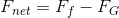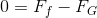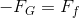The forces of friction and gravity are going to be equal and opposite, causing the drop to remain still on the wall.

### Example Question #3 : Introduction To Forces

How is it possible that two forces of equal magnitude can result in zero net force?

Possible Answers:

The forces are generated in a circle

The forces are parallel to one another

The forces are in opposite directions

The forces are perpendicular to one another

The forces are acting on different objects

Correct answer:

The forces are in opposite directions

Explanation:

If two forces act on a single object, then the net force on the object is equal to the sum of the forces acting on it.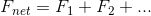Forces are vector quantities, however. This means that all forces have a magnitude and a direction of action. When adding forces, we must take their directions into account. Directions are broken into horizontal and vertical portions for vector addition, and can be positive or negative based on the direction along the axis.

For a net force to be zero, one force must be positive and the other must be negative along a given axis. If the forces are of equal magnitude, then they must be acting in exactly opposite directions in order to cancel each other.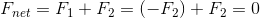### Example Question #4 : Introduction To Forces

Which of the following is not a type of force?

Possible Answers:

Kinetic force

Buoyant force

Friction force

Normal force

Drag (Air resistance)

Correct answer:

Kinetic force

Explanation:

Kinetic force is not a technically correct property. Kinetic energy can be used to generate force, but is not a force in itself. Forces require non-zero acceleration, meaning that they only exist when there is a changein velocity. A change in kinetic energy can indicate a change in velocity, leading to a non-zero force value.

Normal force is the force of a surface upon an object, frequently countering gravity. Friction force is the resistance between an object and a surface, and acts opposite the direction of the object's motion. Buoyant force is the upward force of a fluid on a submerged object. Drag, or air resistance, is a special form of friction force for an object moving through a fluid.

### All High School Physics Resources